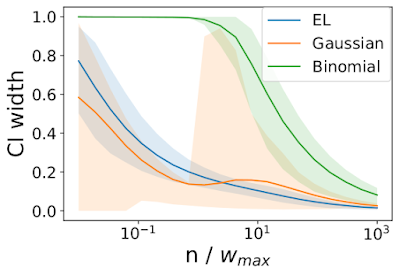## Saturday, December 5, 2020

### Distributionally Robust Contextual Bandit Learning

This blog post is about improved off-policy contextual bandit learning via distributional robustness. I'll provide some theoretical background and also outline the implementation in vowpal wabbit. Some of this material is in a NeurIPS expo talk video, and additional material is in the accepted paper.

## Motivation

In off-policy learning in contextual bandits our goal is to produce the best policy possible from historical data, and we have no control over the historical logging policy which generated the data. (Note production systems that run in closed-loop configurations nonetheless are in practice doing off-policy learning because of delays between inference, training, and model update.) Off-policy learning reduces to optimization of a policy value estimator analogously to supervised learning; however the accuracy of policy value estimation depends upon the mismatch between the policy being evaluated and the policy that generated the data, and therefore can be quite different for different policies (unlike supervised learning, where the differences in estimator resolution across the hypothesis class are less pronounced in practice). To appreciate this effect consider the IPS policy value estimator, $$\hat{V}(\pi; \mu) = \frac{1}{N} \sum_{n \in N} \frac{\pi(a_n|x_n)}{\mu(a_n|x_n)} r_n,$$ where $\mu$ is the historical policy, $\pi$ is the policy being estimated, and our historical data consists of tuples $\{ (x_n, a_n, r_n) \}$. The importance weight $\frac{\pi(a_n|x_n)}{\mu(a_n|x_n)}$ can be quite large if $\pi$ frequently takes an action that $\mu$ rarely takes, causing the estimator to be highly sensitive to a few examples with large importance weights. Even if we initialize learning with $\pi = \mu$, as optimization progresses $\pi$ will induce increasingly different distributions over actions than $\mu$ as the learning algorithm encounters rare events with high reward. To combat this overfitting technique we will introduce regularization.

## Distributionally Robust Optimization

Distributionally robust optimization is a generic method for regularizing machine learning objectives. The basic idea is to consider the observed data as one possible distribution of data (the “empirical distribution”), and then to optimize a worst-case outcome over all distributions that are “sufficiently close” to the empirical distribution. In the case of IPS we can find the smallest policy value estimate over a set of distributions that are close in KL divergence to the empirical distribution, \begin{alignat}{2} &\!\min_{P \in \Delta} & \qquad & \mathbb{E}_P\left[w r\right], \\ &\text{subject to} & & \mathbb{E}_P\left[w\right] = 1, \\ & & & \mathbb{E}_N \left[\log\left( P\right) \right] \geq \phi. \end{alignat} where $w \doteq \frac{\pi(a|x)}{\mu(a|x)}$. It turns out you can do this cheaply (in the dual), and the value of $\phi$ can be computed from a desired asymptotic confidence level. These results follow from classic work in the field of Empirical Likelihood.

The above problem finds a lower bound; finding an upper bound is analogous, resulting in the confidence intervals from the paper:Empirical Likelihood Confidence Intervals are tighter Gaussian intervals.  Not shown: coverage of Empirical Likelihood CIs is better calibrated than Binomial (Clopper-Pearson).

When we do distributionally robust optimization, we are actually optimizing the lower bound on the policy value. The green curve in the above plot is a Clopper-Pearson interval, which does have guaranteed coverage, but is so wide that optimizing the lower bound wouldn't do much until the amount of data is large. The tighter intervals generated by the blue Empirical Likelihood curve imply that lower bound optimization will induce an interesting policy ordering with only modest data.

In practice, even when the empirical mean (empirical IPS value) is fixed, the lower bound is:

• smaller when the policy generates value via few events with importance weights much larger than 1 and many events with importance weights near zero; and
• larger when the policy generates value via many events with importance weights near 1.
This is precisely the kind of regularization we desired. Intuitively, any estimator which is sensitive to a few of the observed examples (aka high leverage) will have a larger penalty because it is “cheap”, as measured by KL divergence, to reduce the probability of those points.

## Implementation in Vowpal Wabbit

To activate the functionality, add the --cb_dro flag to your contextual bandit command line in VW. Note it only effects training, so if you are only predicting you will not see a difference. Hopefully with the default hyperparameters you will see an improvement in the quality of your learned policy, such as in this gist.

Internally VW is solving the lower bound optimization problem from above on every example. There are some modifications:

1. As stated above this would be too expensive computationally, but switching from the KL divergence to the Cressie-Read power divergence allows us to derive a closed form solution which is fast to compute.
2. As stated above the lower bound requires remembering all policy decisions over all time. Instead we accumulate the sufficient statistics for the Cressie-Read power divergence in $O(1)$ time and space.
3. To track nonstationarity we use exponentially weighted moving averages of the sufficient statistics. The hyperparameter --cb_dro_tau specifies the decay time constant.
As always, YMMV.